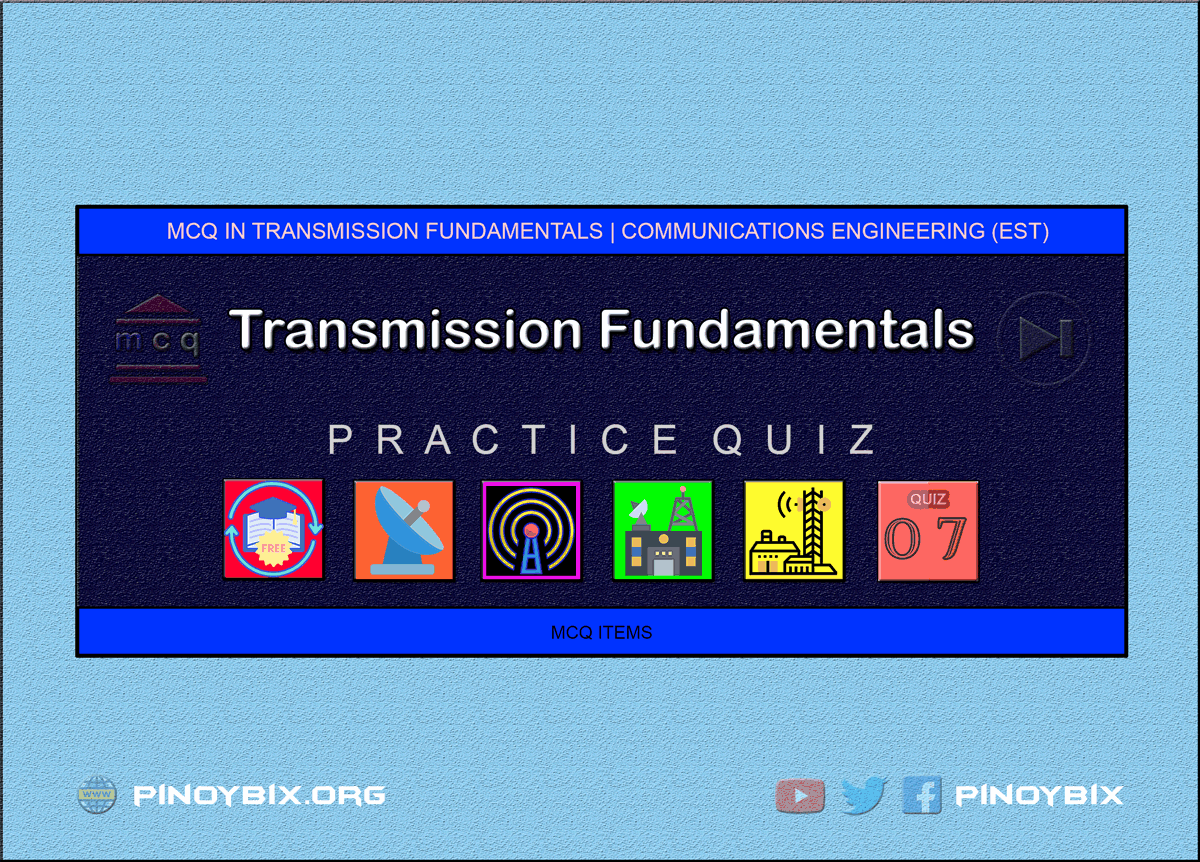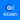# MCQ in Transmission Fundamentals Part 7 | ECE Board Exam

(Last Updated On: May 6, 2020)This is the Multiples Choice Questions Part 7 of the Series in Transmission Fundamentals as one of the Communications Engineering topic. In Preparation for the ECE Board Exam make sure to expose yourself and familiarize in each and every questions compiled here taken from various sources including but not limited to past Board Examination Questions in Electronic System and Technologies, Communications Books, Journals and other Communications References.

#### MCQ Topic Outline included in ECE Board Exam Syllabi

• MCQ in Transmission System
• MCQ in Transmission Medium
• MCQ in Velocity and Line Wavelength
• MCQ in Characteristics Impedance
• MCQ in Primary Line Constants and Propagation Constants
• MCQ in Standing Waves
• MCQ in Voltage Standing Wave Ratio
• MCQ in Telephone Lines and Cables
• MCQ in Wave Guides
• MCQ in Balanced and Unbalanced Lines
• MCQ in Twisted pair wire, Coaxial Cable
• MCQ in Decibel
• MCQ in Signal and Noise Fundamentals

#### Continue Practice Exam Test Questions Part 7 of the Series

301. Category of media with some form of conductor that provides a conduit in which electromagnetic signals are contained.

A. Guided

B. Balanced

C. Unguided

D. Unbalanced

Solution:

302. The conductive connections between elements which carry signals.

B. Antenna

C. Frequency allocations

D. Transmission Lines

Solution:

303. Calculate the characteristic impedance for a line that exhibits an inductance of 4 nH/m and 1.5 pF/m

A. 36.6 ohms

B. 51.6 ohms

C. 22 ohms

D. 24.5 ohms

Solution:

304. Category of media which are wireless

A. Guided

B. Balanced

C. Unguided

D. Unbalanced

Solution:

305. It is a medium or any physical facility used to propagate electromagnetic signals between two locations in a communications system.

A. Transmission medium

B. Channel allocation

C. Frequency allocation

D. Any of these

Solution:

306. It is a metallic conductor system used to transfer electrical energy from one point to another using electrical current flow.

A. Transmitter

B. Multiplexers

D. Transmission line

Solution:

307. A kind of wave where the displacement is in the direction of propagation.

A. Transverse

B. Longitudinal

C. Reverse

D. Perpendicular

Solution:

308. Calculate the capacitance per meter of a 50-ohm load cable that has an inductance of 55 nH/m.

A. 13 pF

B. 18 pF

C. 20 pF

D. 22 pF

Solution:

309. The desirable SWR on a transmission line is

A. 0

B. 1

C. 2

D. Infinity

Solution:

310. A kind of wave where the direction is displacement is perpendicular to the direction of propagation.

A. Transverse

B. Longitudinal

C. Reverse

D. Perpendicular

Solution:

311. A short-circuited half-wavelength line acts like a

A. Parallel resonant circuit

B. Series Resonant Circuit

C. Oscillator

D. LC circuit

Solution:

312. Electromagnetic waves that travel along a transmission line from the source to the load.

A. Reverse waves

B. Transverse waves

C. Incident waves

D. Longitudinal waves

Solution:

313. Electromagnetic waves that travel from the load back toward the source.

A. Incident waves

B. Transverse waves

C. Forward waves

D. Reflected waves

Solution:

314. Sound travels approximately

A. 2200 feet per second

B. 1100 feet per second

C. 550 feet per second

D. 600 feet per second

Solution:

315. Determine the surge impedance for a parallel wire, air dielectric with a ratio of the spacing between conductors and the diameter of 3.

A. 250 ohms

B. 210 ohms

C. 180 ohms

D. 215 ohms

Solution:

316. The rate at which the periodic wave repeats

A. Wavelength

B. Amplitude

C. Period

D. Frequency

Solution:

317. The distance of one cycle occurring in space

A. Wavelength

B. Amplitudes

C. Period

D. Frequency

Solution:

318. Classification of transmission line where both conductors carry current; one conductor carries the signal, the other conductor is the return path

A. Differential transmission lines

B. Unbalanced lines

C. Coaxial cables

D. Balun

Solution:

319. A shorted half-wave line at the operating frequency acts like

A. Capacitor

B. Inductor

C. Series resonant circuit

D. Parallel resonant circuit

Solution:

320. What is the characteristic impedance of a coaxial line, air dielectric with a ratio of the diameter of the outer and inner conductor equal to 1.5?

A. 24.3 ohms

B. 25.6 ohms

C. 13.2 ohms

D. 18 ohms

Solution:

321. Currents that flow in opposite direction in a balanced wire pair is called

A. Longitudinal currents

B. Reverse circuit currents

C. Transverse circuit currents

D. Metallic circuit currents

Solution:

322. Currents that flow in same direction in a balanced wire pair is called

A. Longitudinal currents

B. Reverse circuit currents

C. Transverse circuit currents

D. In-phase currents

Solution:

323. A circuit device used to connect a balanced transmission line to an unbalanced load

A. Slotted lines

B. Stub

C. Balun

D. Quarterwave lines

Solution:

324. The most common type of balun used in relatively high frequency

A. Narrowband

B. Choke

C. Sleeve

D. All of these

Solution:

325. A pattern of voltage and current variations along the transmission line not terminated in its characteristic impedance is called

A. An electric fluid

C. Standing waves

D. A magnetic field

Solution:

326. A type of parallel-conductor transmission lines consists of simply of two parallel wires, closely-spaced and separated by air.

A. Twisted pair

B. Open-wire lines

C. Coaxial cables

D. Twin pair

Solution:

327. A type of parallel-conductor transmission lines which is essentially the same as open-wire transmission line except that the spacers between the conductors are replaced with a continuous solid dielectric that ensures uniform spacing along the entire cable

A. Twisted pair

B. Open-wire lines

C. Coaxial cables

Solution:

328. What is the range of size of wires of a twisted pair?

A. AWG 16 to AWG 26

B. AWG 14 to AWG 25

C. AWG 10 to AWG 20

D. AWG 12 to AWG 28

Solution:

329. What is the characteristic impedance of a coaxial line, polyethylene dielectric with the ratio of the diameter of the outer and the inner conductor of 2.5?

A. 43.5 ohms

B. 23.4 ohms

C. 36.2 ohms

D. 29.8 ohms

Solution:

330. In AWG, the higher the wire gauge ______

A. The higher the diameter and the lower the resistance

B. The smaller the diameter and the higher the resistance

C. The smaller the diameter and the higher the conductance

D. The larger the diameter and the higher the resistance

Solution:

331. Type of twisted pair wire cable that consists of two copper wires where each wire is separately encapsulated in PVC insulation.

A. Shielded twisted pair

C. Unshielded twisted pair

D. Open wire frame

Solution:

332. A shorted quarter-wave line at the operating frequency acts like

A. Series resonant circuit

B. Parallel resonant circuit

C. Capacitor

D. Inductor

Solution:

333. The minimum number of twist for UTP is

A. Two twist per foot

B. Two twist per meter

C. Three twist per foot

D. Three twist per meter

Solution:

334. A delay line using RG-8A/U cable is to exhibit a 5-ns delay. Calculate the required length of the cable.

A. 4.57 ft.

B. 1.23 ft.

C. 6.2 ft.

D. 3.4 ft.

Solution:

335. An open-circuited quarter-wavelength line acts like a

A. Parallel resonant circuit

B. Series resonant circuit

C. Oscillator

D. LC circuit

Solution:

336. Level for category of UTP cable which is suitable only for voice grade telephone signals and very low-speed data applications

A. Level 1 or Cat 1

B. Level 3 or Cat 3

C. Level 2 or Cat 2

D. Cat 4

Solution:

337. An antenna is being fed by a properly terminated two-wire transmission line. The current in the line at the input end is 3 A. The surge impedance of the line is 500 ohms. How much power is being supplied to the line?

A. 3.1 kW

B. 2.5 kW

C. 1.6 kW

D. 4.5 kW

Solution:

338. Level or Category of UTP cable which was developed for IEEE 802.5 token ring local area networks operating at a transmission rates of 4Mbps

A. Level 1 or Cat 1

B. Level 3 or Cat 3

C. Level 2 or Cat 2

D. Cat 4

Solution:

339. If the period of one complete cycle of a radio wave is 0.000001 s, what is the wavelength?

A. 300 m

B. 200 m

C. 100 m

D. 400 m

Solution:

340. If the two towers of a 950-kHz antenna are separated by 120 electrical degrees, what is the tower separation in feet?

A. 231 ft.

B. 235 ft.

C. 176 ft.

D. 345 ft.

Solution:

341. Category of UTP used for virtually any voice or data transmission rate up to 16 Mbps, has a minimum of 3 turns per inch.

A. Category 5e

B. Category 4

C. Category 5

D. Category 3

Solution:

342. The mismatch between the antenna and transmission line impedances cannot be corrected for by

A. Using an LC matching network

C. Using a balun

D. Adjusting the length of transmission line

Solution:

343. Category of UTP that was designed for data transmission rates up to 20 Mbps

A. Category 5e

B. Category 4

C. Category 5

D. Category 3

Solution:

344. Variation of CAT5 cables that are intended for data transmission rates up to 250 Mbps

A. Category 5e

B. Category 2

C. Category 6

D. Category 3

Solution:

345. A type of twisted-pair wherein its wires and dielectric are enclosed in a conductive metal sleeve called a foil.

A. STP

C. UTP

Solution:

346. It is the name given to the area between the ceiling and the roof in a single-story building or between the ceiling and the floor of the next higher level in a multistory building.

A. Attic

B. Rooftop

C. Plenum

D. Ceiling

Solution:

347. It consists of center conductor surrounded by dielectric material, then a concentric shielding, and an environmental protection outer jacket.

A. Twisted pair

B. Coaxial cable

D. Open wire

Solution:

348. In a transmission line, it refers to the woven stranded mesh or braid that surround some types of coaxial cables

A. Grounding

B. Shielding

C. Degaussing

D. Any of these

Solution:

349. A coaxial cable with one layer of foil insulation and one layer of braided shielding.

A. Backup shielding

B. Temporary shielding

C. Dual shielding

D. Interference shielding

Solution:

350. At very high frequencies, transmission lines are used as

A. Tuned circuits

B. Antennas

C. Insulators

D. Resistors

Solution:

#### Questions and Answers in Transmission Fundamentals Series

Following is the list of multiple choice questions in this brand new series:

MCQ in Transmission Fundamentals
PART 1: MCQ from Number 1 – 50                        Answer key: PART 1
PART 2: MCQ from Number 51 – 100                   Answer key: PART 2
PART 3: MCQ from Number 101 – 150                 Answer key: PART 3
PART 4: MCQ from Number 151 – 200                 Answer key: PART 4
PART 5: MCQ from Number 201 – 250                 Answer key: PART 5
PART 6: MCQ from Number 251 – 300                 Answer key: PART 6
PART 7: MCQ from Number 301 – 350                 Answer key: PART 7
PART 8: MCQ from Number 351 – 400                 Answer key: PART 8
PART 9: MCQ from Number 401 – 450                 Answer key: PART 9
PART 10: MCQ from Number 451 – 500                Answer key: PART 10

### Complete List of MCQ in Communications Engineering per topic

Help Me Makes a Difference!

 P inoyBIX educates thousands of reviewers/students a day in preparation for their board examinations. Also provides professionals with materials for their lectures and practice exams. Help me go forward with the same spirit. “Will you make a small gift today via GCASH?”+63 966 459 6474 Option 1 : \$1 USD Option 2 : \$3 USD Option 3 : \$5 USD Option 4 : \$10 USD Option 5 : \$25 USD Option 6 : \$50 USD Option 7 : \$100 USD Option 8 : Other Amount© 2014 PinoyBIX Engineering. © 2019 All Rights Reserved | How to Donate? |